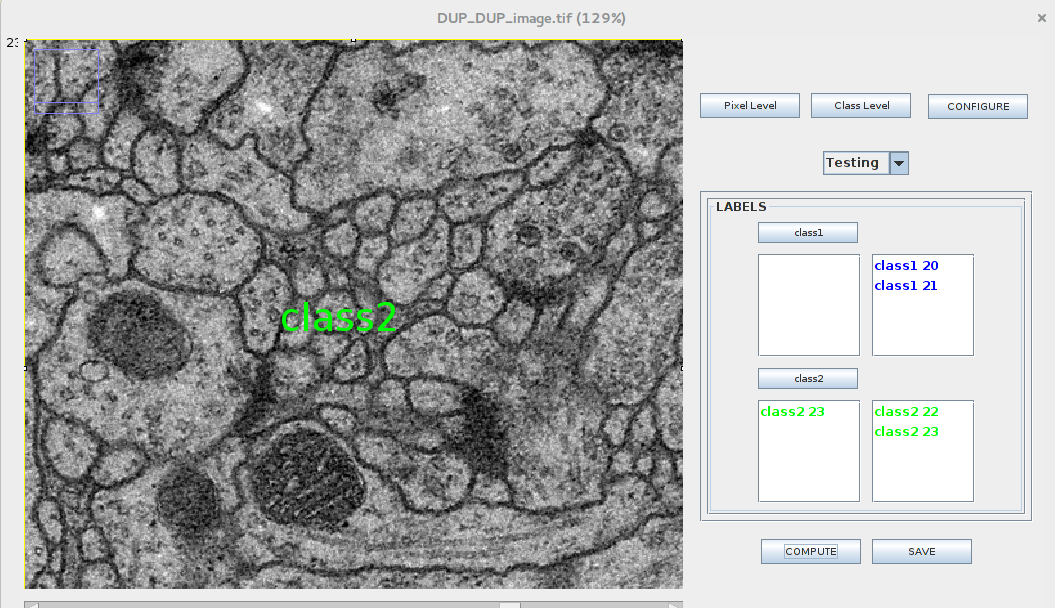# Developers

Student: Mukesh Gupta
Mentor: Dimiter Prodanov Sumit Kumar Vohra

# Project description

This project is about to provide the general purpose environment that allows biologists and other domain experts to use transparently state-of-the-art techniques in machine learning to improve their image segmentation results.
ImageJ is a public domain Java image processing program extensively used in life sciences. Active Segmentation plugin is the redesign of existing Trainable Weka Segmentation (TWS) of ImageJ. The main functionality of the platform was already developed in the context of GSOC 2016 but the platform is still under development. In last Google summer of code, the major focus was on integrating generic filter families and specifically on one family of filters i.e. Gaussian Scale Space. The Active Segmentation provides generic functionality and user friendly interface so that the user can include the state of the art filters and machine learning frameworks from the WEKA library:

• Active learning.
• Multi-instance learning designed by third party in a robust manner
In this Google summer of code we expanded the existing functionality of the Active Segmentation plugin. Earlier this tool only used for Segmentation, now functionality has been extended to classification of the images. We used Zernike Moments as a feature vector so we extracted zernike moments from the images and then used these features to train our SVM classifier to classify images.
Zernike moments is the set of orthogonal Zernike polynomials defined over the polar coordinate space inside a unit circle. The two-dimensional Zernike moments of order p with repetition q of an image intensity function $f(r, \theta)$ are defined as
$Z_{pq}= \frac{p+1}{\pi} \int_{0}^{1} \int_{-\pi}^{+\pi} V_{pq}(r,\theta)rdrd\theta,\; |r| \le 1$
where p order Zernike polynomials are defined as $V_{pq}(r, \theta) = R_{pq}(r)e^{-jq\theta},\; j=\sqrt{-1}$
and the real-valued radial polynomials defined as $R_{pq} = \sum_{k=0}^\frac{p-|q|}{2} (-1)^2 \frac{(p-k)!}{k!(\frac{p+|q|}{2}-k)!(\frac{p-|q|}{2}-k)!}r^{p-2k}$
where $0\le|q|\le p,\; p-|q|\; is\;even,\; p\ge0$
It can be seen from the above formulae that Computation of Zernike radial polynomials will takes too much time when order goes high, so we have decided to use other recursive formula to compute Zernike radial polynomials $R_p^q(r) = r[R_{p-1}^{|q-1|}(r)+R_{p-1}^{q+1}(r)]-R_{p-2}^q(r)$
This Formulae has been taken from this paper

# Filter Framework

Filter Framework is responsible for automatic loading of filters. It automatically finds all JAR files in the path and first level of subdirectories and those have implemented "IFilter" interface.

Active Segmentation Filters Screen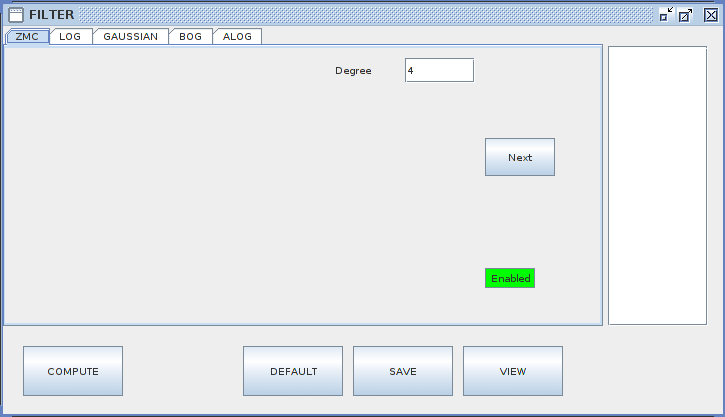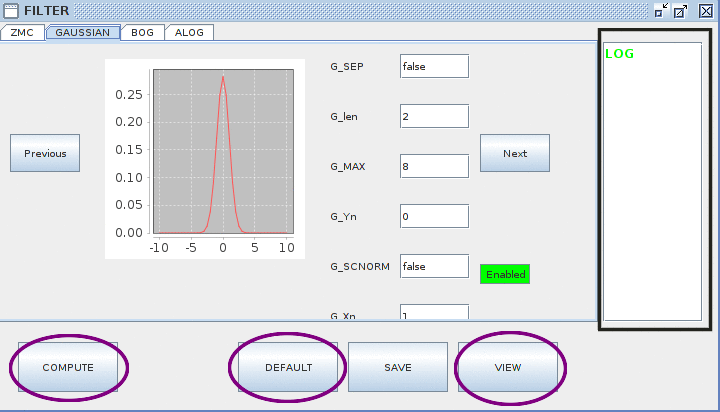• The right black marked box is used to enable the unselected filters. User need to double click on filter name to enable it
• The settings for each filter is provided in the centre along with the shape of filter
• Filters process is started using the "COMPUTE" button.
• Filters meta data is dumped using the "SAVE" button.
• The "View" button is used to view the filters result for each image slice.
• The "Default" button is used to set the set default settings for the filter as provided by the developers .

Earlier filter was applying on each slice of imageStack one by one which takes time for ALOG, BOG, LOG and GAUSSIAN is around 27 seconds but after applying filter on each slice parallely it comes down to 17 seconds.

# Feature Screen

Feature Screen of the active segmentation is very similar to the TWS(Trainable Weka Segmentation). It will start with two classes i.e Binary Classification

This screen showing the Class level pixel classification

User selects images which can be used for training purpose.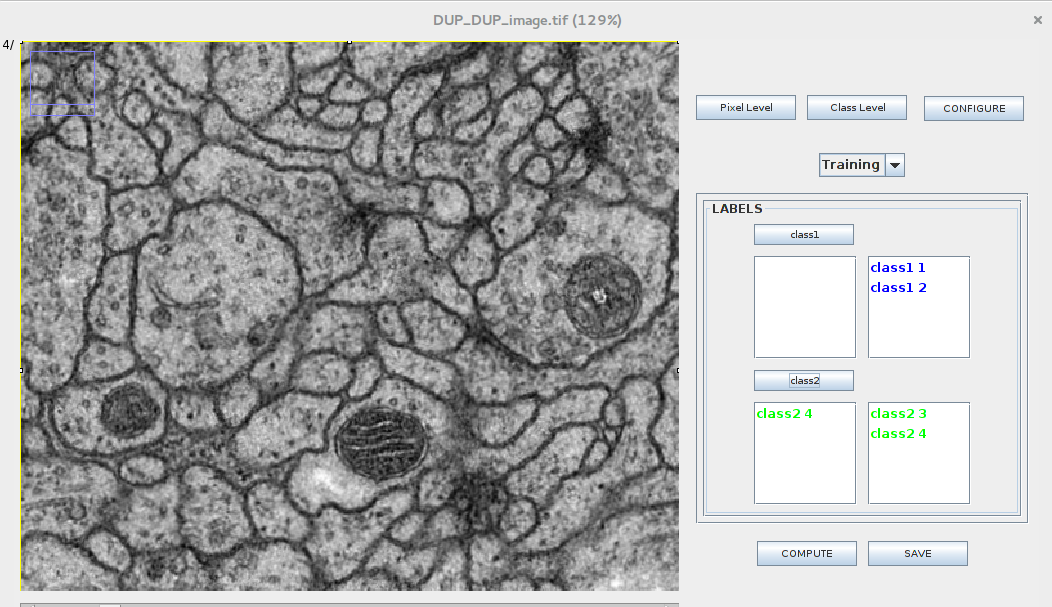User selects images which can be used for testing purpose.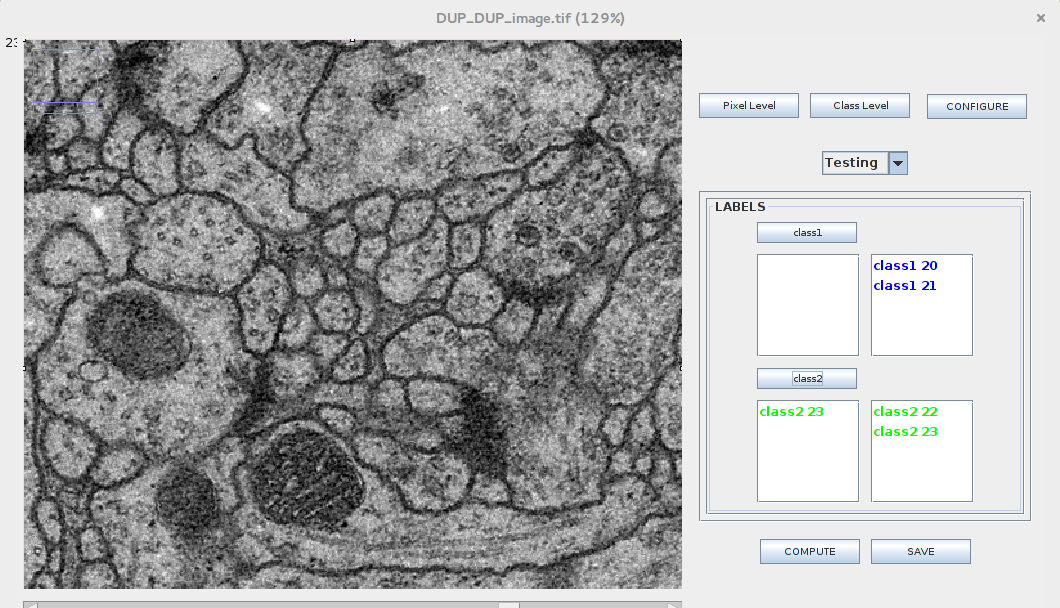Classification Result would be show on top of the image.